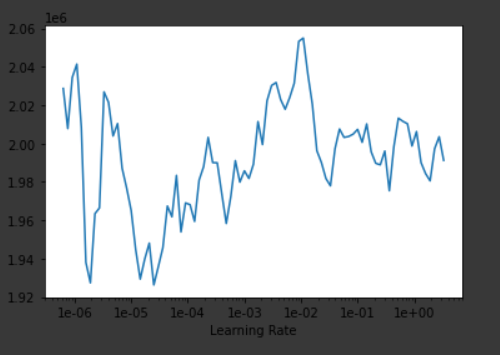# Fastai.Tabular

Im trying to do a regression problem with 1500 rows but it’s not working for some reason. If anyone can tell me what I’m doing wrong, that would be really helpful.
This is my code:

data = (TabularList.from_df(train, path=’.’, cat_names=cat_names, cont_names=cont_names, procs=procs)
.split_by_idx(list(range(1200,1500)))
.label_from_df(cols = dep_var, label_cls=FloatList)
.databunch())
data.show_batch(rows=10)

# Create deep learning model

learn = tabular_learner(data, layers=[1000,250], metrics=rmse,
ps=[0.001,0.01], emb_drop=0.02,
callback_fns=ShowGraph)

learn.lr_find()

learn.recorder.plot()learn.fit_one_cycle(10, max_lr=slice(3e-02), wd=0.1)